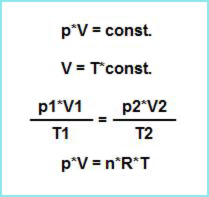Knowledge database: Stoichiometry: Gases The state of a gas is defined by its temperature, pressure and volume. Here we must also mention the conditions at which a gas is in the, so called, standard state - a pressure of 101.325 kPa and a temperature of 273.15 K (or 0 °C). Every change of the state of a gas happens according to gas laws which were mentioned in earlier chapters. Although these laws are valid for "ideal" gases, they can be used for real gases as well, since, at the normal laboratory conditions of pressure and temperature, the deviation isn't large enough to cause any significant problems. Here we will mention again all the laws related to gases that are important in stoichiometry, in form of mathematical formulas:(1) Boyle-Marriote's law can be expressed by the formula: p*V = constant. (2) Gay-Lussac's law: V = T*constant. (3) By combining Boyle-Marriote's law and Gay-Lussac's laws, we get the following important formula, which shows us the mathematical relations between pressure, temperature and volume (at a constant amount of gas): (p1*V1)/T1 = (p2*V2)/T2. (4) Ideal gas equation: p*V = n*R*T.